# Week 10: Reducibility¶

## Tuesday¶

Last time, we started to use the undecidability of $A_{\textsf{TM}}$ to prove the undecidability of other languages, starting with the halting problem. Today, we generalize this approach to more languages.

Today our decidability proofs are about some sort of "program", which might be a Turing machine, a Python program, or some other more exotic sort of computing device. It may or may not read an input string.

We want to show that it is undecidable whether some program $P$ has some property $\phi$. We want to do so by reduction from $A_{\textsf{TM}}$; sometimes the reduction is from some other language (e.g., Theorem 5.4), but $A_{\textsf{TM}}$ is really very useful and it will be $A_{\textsf{TM}}$ most of the time.

The proof goes like this: Assume that there is some TM $R$ that can decide whether program $P$ has property $\phi$. Then, we want to use $R$ to implement a "universal decider" $S$, that is, a TM that decides $A_{\textsf{TM}}$. That implementation usually has three steps:

$S =$ "On input $\langle M, w\rangle$:

1. Convert $\langle M, w\rangle$ into a program $P$.
2. Run $R$ on $P$.
3. If $R$ accepts, accept; if $R$ rejects, reject.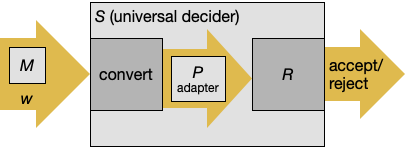Let me say a little more about these three steps in reverse order.

In our reduction from $A_{\textsf{TM}}$ to the halting problem, step 3 was more complex than this. But usually it's very simple, like the above. (In fact, there is a more restricted notion of reduction (Section 5.3) that requires that step 3 to be the above.) Sometimes (like Theorem 5.2 below), it will be flipped: If $R$ accepts, reject; if $R$ rejects, accept.

Step 2 is always "Run $R$ on $P$", without exception as far as I know.

In our reduction from $A_{\textsf{TM}}$ to the halting problem, step 1 was trivial ($\langle M, w \rangle$ maps to itself), but in general, step 1 really has to do something. It has to change the TM+string $\langle M, w\rangle$ into $P$, which acts as an "adapter" between the property we want to detect (whether $M$ accepts $w$) into the property that $R$ able to detect ($\phi$).

When $P$ is another Turing machine, step 1 involves creating a third TM besides $R$ and $S$. Since it can be hard to keep track of so many TMs, I think it's helpful to start with an example problem about something other than Turing machines.

### A problem about Python programs¶

Let's show that it is undecidable whether a given Python program $P$ writes to the filesystem. It would be great for security if this were decidable, but unfortunately it's not.

Suppose, for the sake of contradiction, that this is decidable. That is, there exists a TM $R$ that accepts a Python program $P$ if and only if $P$ would write to the filesystem. Let's call it the "write-detector."

We're going to build a universal decider $S$ that somehow uses the write-detector $R$ to decide $A_{\mathsf{TM}}$. We can't feed $\langle M, w\rangle$ to the write-detector $R$ because $R$ wants a Python program. So we need to convert $M$ and $w$ into a Python program.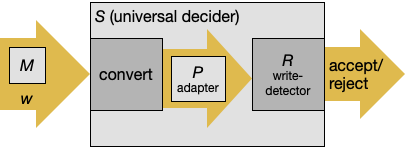We assume a Python function simulate that simulates a TM and returns True for accept or False for reject; this function can also potentially loop. It should be obvious that such a function exists, although it's long enough that we don't bother to write it out. Then the universal decider can be implemented as $S =$ “On input $\langle M, w\rangle$:

1. Construct the Python program, called $P$:
 import os
if simulate(M, w):
os.system("rm -rf *")
where M and w are filled in with data structures representing $M$ and $w$, respectively.
2. Run $R$, the write-detector, on $P$.
3. If $R$ detected a write, then accept.
4. Otherwise, reject.

To see that $S$ is a universal decider, let's walk through the possible cases:

• If $M$ accepts $w$, then $P$ would wipe out your files. $R$ detects this, and so $S$ accepts.
• If $M$ rejects $w$, then $P$ does not wipe out your files. $R$ does not detect any writes, and so $S$ rejects.
• Similarly, if $M$ loops on $w$, then $P$ would run forever but would not wipe out your files. $R$ does not detect any writes, and so $S$ rejects.

Thus $S$ decides $A_{\mathsf{TM}}$ as desired, which is a contradiction. We conclude that it's undecidable whether a given Python program writes to the filesystem.

In general, when you're trying to prove that detecting property $\phi$ is undecidable, the program $P$ usually has to do the following things:

• Simulate $M$ on $w$.
• If $M$ accepts, then exhibit property $\phi$.
• If $M$ rejects, then don't exhibit property $\phi$.
• You must also set it up so that if $M$ loops, property $\phi$ is not exhibited.

Question. Try Problem 5.12: Show that it is undecidable, given a Turing machine $M$, whether there is any input $w$ for which $M$ ever erases a symbol (that is, overwrites a non-blank symbol with a blank symbol).

### Properties of languages¶

Read Theorems 5.2–4 and their proofs (pages 217–220).

The next two theorems, 5.2 and 5.3, are a little different because they are not properties of a Turing machine and an input; they are properties of the whole language that a Turing machine recognizes. But the general scheme of their proofs is the same.

Here, we walk through the proof of Theorem 5.2. It says that there is no "emptiness-detector" TM that decides whether another TM recognizes an empty language.

Suppose that there $R$ is an emptiness detector. Then we need to implement $S$, a universal decider.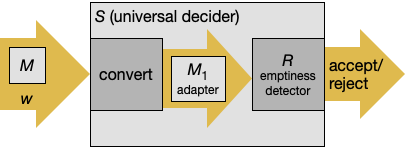Given $M$ and $w$, we "adapt" them into a machine $M_1$ that recognizes a nonempty language iff $M$ accepts $w$. We're going to construct $M_1$ differently from the book; if you like the book's version better, that's fine too.

$M_1 =$ “On input $x$:

1. Run $M$ on input $w$.
2. If $M$ accepts $w$, then accept $x$.
3. If $M$ rejects $w$, then reject $x$.

Let's think carefully about what $M_1$ would do.

• If $M$ accepts $w$, then $M_1$ accepts all strings $x$. That is, $M_1$ recognizes $\Sigma^\ast$, a nonempty language.
• If $M$ rejects $w$, then $M_1$ rejects all strings $x$. That is, $M_1$ recognizes $\emptyset$.
• If $M$ loops on $w$, then $M_1$ also loops on all strings $x$. So, $M_1$ recognizes $\emptyset$.

Finally, we define a universal decider $S =$ “On input $\langle M, w\rangle$:

1. Construct $M_1$ as described above.
2. Run $R$, the emptiness-detector, on $M_1$.
3. If $R$ detected an empty language, reject.
4. Otherwise, accept.

Here's how $S$ works:

• If $M$ accepts $w$, then $M_1$ would recognize $\Sigma^\ast$, a non-empty language, and so $S$ accepts.
• If $M$ rejects or loops on $w$, then $M_1$ would recognize $\emptyset$, and so $S$ rejects.

Thus $S$ decides $A_{\mathsf{TM}}$ as desired, which is a contradiction. We conclude that it's undecidable whether a given Turing machine recognizes the empty language.

We've tried to follow a general recipe for proving undecidability results. It turns out that this recipe can be distilled into a catch-all theorem called Rice's Theorem: any property of a language recognized by a Turing machine, as long as the property is not always true or always false, is undecidable.

There are some properties of the internal working of a Turing machine that are decidable (you'll see one in HW7). But broadly speaking, questions about external behaviors of computer programs tend to be undecidable. When you see problems like this in real life -- and you definitely will -- you should smell undecidability and think twice before trying to implement a solution to the problem.

### Other undecidable problems¶

Sometimes, the existence of a Turing machine simulator is not so obvious, and we have to think more carefully about it.

Question. An unrestricted grammar is like a CFG, but the left-hand sides can be strings. A derivation starts with $S$ and continues until no more rules can be applied. Show that it is undecidable whether an unrestricted grammar generates a nonempty language. Hint: Convert $\langle M, w\rangle$ to a grammar whose starting rule generates the start configuration: $$S \rightarrow q_0 w$$ and for each transition of $M$, create a grammar rule (or rules) to simulate that transition.

See the survey by Poonen for more examples of undecidable problems.

## Thursday¶

Today we make good on a claim we made on the first day of class: There is no such thing as a computer program that can solve Post's Correspondence Problem (which we demonstrated as the game Poco).

Last time, we laid out the following general outline of an undecidability proof, in which we implement a universal decider depicted like this:Moreover, we said that the way $P$ usually works is by simulating $M$ on $w$. That works if $P$ is a Turing machine or Python program or some other thing that is as powerful as a Turing machine. But what if $P$ is something simpler, like an instance of PCP?

### Undecidable search problems¶

Read the subsection "Reductions via Computation Histories" up to Theorem 5.9 and its proof.

When you have to prove an undecidability result about a thing more limited than a Turing machine and involving a "there exists," a very useful technique for simulating a Turing machine is to use computation histories.

Recall that a configuration can be encoded as a string as described on page 168: $uqav$ is the configuration where $u$ is the tape to the left of the head, $q$ is the current state, $a$ is the symbol under the head, and $v$ is the tape to the right of the head.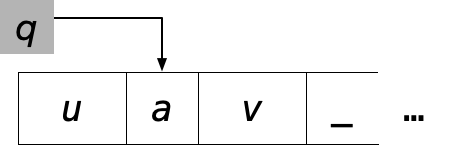A computation history is a sequence of configurations $C_1\texttt{#}C_2\texttt{#}\cdots\texttt{#} C_l$, where $C_1$ is a start configuration and each $C_{i-1}$ yields $C_i$. An accepting computation history is one whose last configuration is an accepting configuration.

The reason computation histories are interesting is that an accepting computation history for $w$ may be easier to recognize than $w$ itself.

### LBA emptiness¶

The book's first example of a proof using computation histories involves linear bounded automata, which are just Turing machines where the tape is exactly as long as the input string. For example, if the input string is $\texttt{0110}$, an LBA looks something like: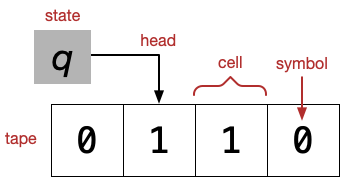Note that there are no cells after the input string. (LBAs are interesting because they recognize a class of languages, the context-sensitive languages, that are another rung on the Chomsky hierarchy that we haven't talked about before. The CSLs are also generated by context-sensitive grammars, whose rules are of the form $\alpha B \gamma \rightarrow \alpha \beta \gamma$ where $\alpha$, $\beta$, and $\gamma$ are strings of terminals and nonterminals.)

We want to prove that it is undecidable whether an LBA recognizes the empty language. Up till now, our proofs have tried to implement a universal decider $S$ that takes input $\langle M, w\rangle$ and builds an "adapter" $B$ that somehow involves simulating $M$ on $w$.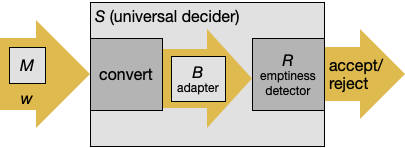But here our "adapter" has to be an LBA, and an LBA isn't powerful enough to simulate $M$. But, an LBA is powerful enough to test whether its input is an accepting computation history for $M$ on $w$. And the emptiness-detector $R$ is able to test whether there exists something that $B$ accepts, which means that there exists an accepting computation history for $M$ on $w$, which means that $M$ accepts $w$.

Read the rest of Section 5.1 (pages 223–226).

The core of the proof is writing the LBA that checks whether its input is an accepting computation history for $M$ on $w$. It's not too hard to imagine (I think) how a TM could do this without requiring any scratch space beyond the end of the input string.

Then we can implement the universal decider $S =$ "On input $\langle M, w\rangle$":

1. Construct an LBA $B$ that accepts accepting computation histories for $M$ on $w$.
2. Run $R$ on $B$.
3. If $R$ accepts, reject; if $R$ rejects, accept.

As before, let's think through the various possible outcomes:

• If $M$ accepts $w$, then there exists a computation history for $M$ on $w$, so $B$ recognizes a non-empty language, so $R$ rejects $B$, so $S$ accepts $\langle M,w\rangle$.
• If $M$ rejects or loops on $w$, then there does not exist a computation history for $M$ on $w$, so $B$ recognizes the empty language, so $R$ accepts $B$, so $S$ rejects $\langle M,w\rangle$.

### Post's Correspondence Problem¶

Recall that an instance of PCP is a set of "dominos", for example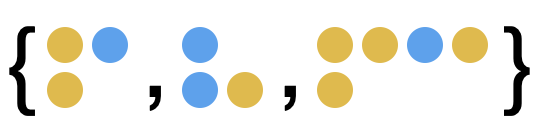and to solve an instance, you must find a sequence of dominos such that the top row equals the bottom row.

In the first class, we demonstrated a gamified version of PCP called Poco.

PCP appears too simple to simulate a Turing machine, but note that solving PCP is a search problem: we want to know whether there exists a solution.

To prove that PCP is undecidable, the book introduces a slightly modified version of PCP (MPCP), which has a special domino that the solution must start with. The book proves that MPCP is undecidable by reduction from $A_{\mathsf{TM}}$, then proves that PCP is undecidable by reduction from MPCP.

The proof constructs an MPCP instance $P$ such that $P$ has a solution iff $M$ accepts $w$. The solution must start with the domino in Part 1: $$\left[ \frac{\#}{\#q_0 w_1 \cdots w_n \_ \#} \right]$$ After putting down this first domino, the bottom row is longer than the top row. Thereafter (as you can convince yourself by studying the dominos), the top row can never become longer than the bottom row. This property is convenient because it allows us to think of the excess portion of the bottom row as a queue: a domino $\left[ \frac{t}{b} \right]$ can be thought of as "pop $t$ from the left and push $b$ on the right". For example,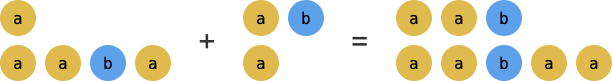In this picture, the excess is initially $\texttt{aba}$. Adding the domino $\left[ \frac{\texttt{ab}}{\texttt{a}} \right]$ acts like popping $\texttt{ab}$ on the left and pushing $\texttt{a}$ on the right, making the excess $\texttt{aa}$.

With that in mind, perhaps we can interpret the dominos in the construction more easily. The domino in Part 1 (shown above) pushes the start configuration followed by $\#$.

Parts 2–5 work together to pop a configuration and push possible successor configurations. I modified them a little bit:

\begin{align*} (4,5\text{a}) \qquad &\left[ \frac{a}{a} \right] && \text{for each $a \in \Gamma \cup \{\#\}$} \\ (2) \qquad &\left[ \frac{qa}{br} \right] && \text{for each transition $q \xrightarrow{a \rightarrow b, \text{R}} r$} \\ (2') \qquad &\left[ \frac{qa\#}{br\_\#} \right] && \text{for each transition $q \xrightarrow{a \rightarrow b, \text{R}} r$} \\ (3) \qquad &\left[ \frac{cqa}{rcb} \right] && \text{for each transition $q \xrightarrow{a \rightarrow b, \text{L}} r$ and $c \in \Gamma$} \\ (3') \qquad &\left[ \frac{\#qa}{\#rb} \right] && \text{for each transition $q \xrightarrow{a \rightarrow b, \text{L}} r$} \end{align*}

Domino (4,5a) just copies symbols that don't change from one configuration to the next. Domino (2) handles move-right rules; (2$'$) handles the case where the head is at the right end of the explored part of the tape. Domino (3) handles move-left rules; (3$'$) handles the case where the head is at the left end of the tape.

Parts 6–7 work together with Part 4,5a to recognize an accepting configuration, and when it is found, to eat it away little by little until there is nothing left, at which point the solution is complete.

\begin{align*} (6\text{a}) \qquad &\left[ \frac{a q_{\text{accept}}}{q_{\text{accept}}} \right] \\ (6\text{b}) \qquad &\left[ \frac{q_{\text{accept}} a}{q_{\text{accept}}} \right] \\ (7) \qquad &\left[ \frac{q_{\text{accept}} \# \#}{\#} \right] \\ \end{align*}

### Halt¶

That's it for Unit III of the course! We've seen:

• Turing machines (TMs)
• What they are
• How to write them
• Church-Turing thesis
• TMs define what it means to compute
• Variants of TMs don't make TMs more powerful
• TMs can even simulate any TM given to it
• Undecidability
• Almost all languages are undecidable
• We can prove this by diagonalizability
• Or we can prove it by reduction from another undecidable language

In the next and final unit, we will explore the time complexity of deciding languages using Turing machines, and whether some languages are inherently difficult to decide.